# Sum to Infinity

The sum to infinity of a sequence is the sum of an infinite number of terms in the sequence.It is only possible to compute this sum if the terms of a sequence converge to zero. Even then, it is not always possible. This is called a necessary but not sufficient condition – for a sum to infinity to be defined, the sequence must converge to zero – but not all sequences that converge to zero have a defined sum to infinity. We will see on this page that it is possible to compute this sum for all geometric sequences that have a common ratio.

For any sequence that diverges, the sum of the sequence also diverges.

The arithmetic sequence 4, 7, 10, 13, 16, … diverges

The series 4 + 7 + 10 + 13 + 16 also diverges.

# Sum to Infinity of a Geometric Sequence

Whenand, then the sequence converges to zero, regardless of the first term (Althoughdoesn’t generate a very interesting sequence). Let’s examine the sum to infinity of a couple of examples, then generalise. First, we need to be OK with the following two ideas:

Concept 1: Multiply a fraction byNotice that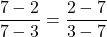Both sides in the line above are equal to positive.

When we multiply any value by(the multiplicative identity) we do not change its value. The fraction. Let’s begin with the left hand side and multiply by.We do this in order to rearrange our summation formula for a geometric series as follows:This version of the summation formula is easier to use when.

Concept 2: Ifthen asTake any number that is less than one. For example,.

On the applet below, increase the value ofto observe that asincreases, the value ofdecreases towards zero.

This applet offers 15 decimal places, however, if you considerwe know that this number is not zero. Very, very small, but not zero.

We say ‘tends to’ when a value that is changing approaches another value, which may be a constant or a function. We use the notation ‘‘ for ‘tends to’.

Therefore if, then as,.

The line above reads ‘if the absolute value of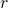is less than 1, then astends to infinity,tends to zero.’

Another way to write this is: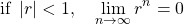the line above reads: ‘If, the limit ofastends to infinity, is zero’.

# Example

When adding together an infinite number of terms of a geometric sequence that has, it would seem that there is a limit.

Consider the sequence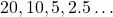Here is the sum of the first several terms:It would appear that asgets larger,gets closer to 40.

According to the summation formula for a geometric sequence, the sum of the firstterms is:However, asapproaches infinity,approaches zero.

Therefore, the limit of this sum isWe say# Conclusion

In general, becauseas, we replacewith zero in the formula: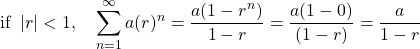That is:## Example

A geometric sequence begins. Calculate the sum to infinity of this sequence.

In this case,,. Therefore,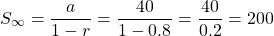# Practice

Try these problems on the CK12 website on summing infinitely many terms of a geometric sequence.